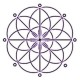# Chaitanya's Random Pages

## July 7, 2011

### The number 3456

Filed under: mathematics — ckrao @ 10:07 pm

Here is a cute numerical fact that I did not know before. Not only is the number 3456 easy to remember in having its digits in arithmetic sequence, but its smallest prime factor is particularly small:

#$\displaystyle 3456 = 2^7 \times 3^3$

That’s right, its only prime factors are 2 and 3. 🙂 (See OEIS A033845 for other numbers of this form.) One can easily read off that it has 32 factors. It is by no means the smallest integer to have this many factors (that honour belongs to 840), but it is the smallest to have 30 non-prime factors (see OEIS A055079). The sum of the cubes of its digits is 27 + 64 + 125 + 216 = 432, which curiously is a factor of 3456 (OEIS A034088).

Halving this number gives$1728 = 12^3$ which is one less than the Hardy-Ramanujan number 1729, the smallest number that can be written as the sum of two positive cubes in two different ways. Having known this previously, I guess I should have seen earlier in my life that 3456 is double 1728 and therefore has small prime factors!

Finally, using the above factorisation and knowing that 128 = 101 + 27 allows us to write 3456 in this attractive form (see OEIS A105814):$\displaystyle 3456 = 27\times 27 + 2727$

1.🙂 Please Could you explain this?

“Finally, using the above factorisation and knowing that 128 = 101 + 27 allows us to write 3456 in this attractive form 3456 = 27\times 27 + 2727”

THanks!

Comment by Uni — March 7, 2015 @ 10:48 pm

•Yes,$3456 = 128 \times 27 = (27+101) \times 27 = 27\times 27 + 101 \times 27 = 27\times 27 + 2727$.

Comment by ckrao — March 8, 2015 @ 12:49 am

•Thanks!! Now I remember that school lesson 🙂 hehe

Comment by Uni — March 8, 2015 @ 10:05 pm

Create a free website or blog at WordPress.com.